# Plane

On how many parts divide plane 6 parallels?

Correct result:

n =  7

#### Solution:

$n = 6+1 = 7$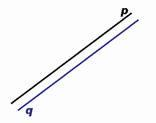We would be very happy if you find an error in the example, spelling mistakes, or inaccuracies, and please send it to us. We thank you!## Next similar math problems:

• Line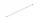How many parts of line divide 5 (different) points that lie on it?
• CakesOn the bowl were a few cakes. Jane ate one-third of them, Dana ate a quarter of those cakes that remained. a) What part (of the original number of cakes) Dana ate? b) At least how many cakes could be (initially) on thebowl?
• Math classificationIn 3A class are 27 students. One-third got a B in math and the rest got A. How many students received a B in math?
• The resultHow many times I decrease the number 1632 to get the result 24?
• Ten pupils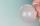10 pupils went to the store. 6 pupils bought lollipops and 9 pupils bought chewing gum. How many pupils have bought both lollipops and chewing gums (if everyone bought something)?
• The number 3Ski organizers should print the start numbers from 1 to 45. How many times will they use the number 3 when printing?Added together and write as decimal number: LXVII + MLXIV
• CagesHonza had three cages (black, silver, gold) and three animals (guinea pig, rat and puppy). There was one animal in each cage. The golden cage stood to the left of the black cage. The silver cage stood on the right of the guinea pig cage. The rat was in th
• Eq1Solve equation: 4(a-3)=3(2a-5)
• Trip to MoraviaSixth grade went on a trip to Moravia. Each of the 26 pupils paid CZK 320 and the school paid a total of CZK 3,510. What was the average price of a trip per student?
• Six te 2If 3t-7=5t, then 6t=
• Circles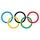For the circle c1(S1; r1=146 cm) and c2(S2; r2 = 144 cm) is distance of centers |S1S2| = 295 cm. Determine the distance between the circles.
• One frame5 picture frames cost € 12 more than three frames. How much cost one frame?
• Valid numberRound the 453874528 on 2 significant numbers.
• Equation with one variableSolve the following equation with one unknown: 5(7s + 5) =130
• If-then equationIf 5x - 17 = -x + 7, then x =
• Expressions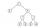Find out value of expressions if a = -1, b =2: x=b - 2a - ab y=a3 - b2 - 2ab z=a2 b3 - a3 b2 w=a + b + a3 - b2